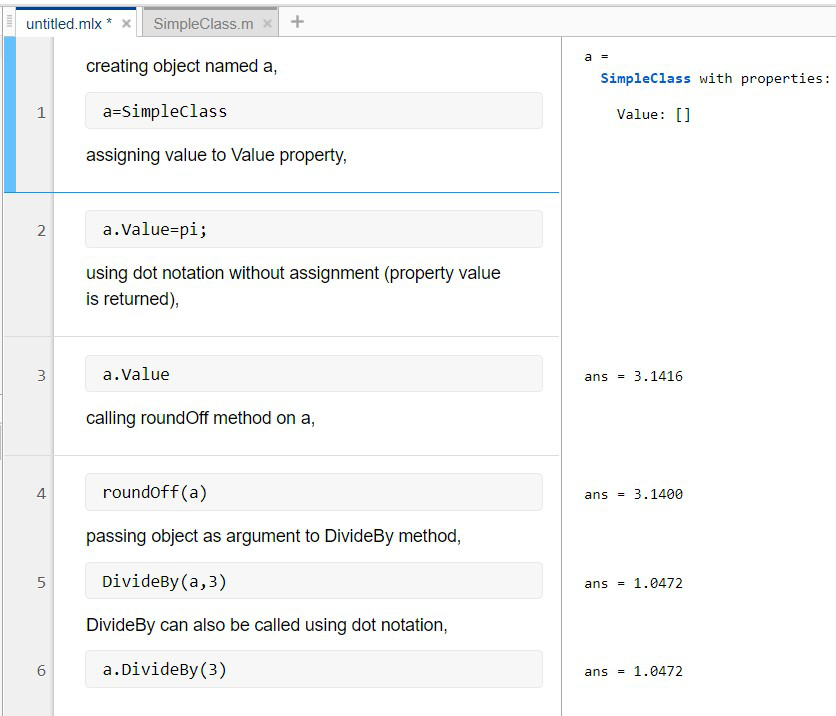Related Articles

# Classes and Object in MATLAB

• Last Updated : 09 May, 2021

A class is a blueprint that defines the variables and the methods which provide a commonly shared basis for its corresponding objects. It defines an object that encapsulates data and the operations performed on that data. classdef is a keyword used to define MATLAB classes.

Syntax to define a class:

```classdef (Attributes) ClassName < SuperclassName

properties (Attributes)
PropertyName
PropertyName size class {validation functions}
end

methods (Attributes)
function obj = methodName(obj,arg2,...)
...
end

end

events (Attributes)
EventName
end

end```

### Components of MATLAB class:

MATLAB class has three major components (which are MATLAB functions used to query the respective class members for a given object or class name):

• Properties blocks: Define the properties that store data for each of the objects of the class.
• Methods blocks: Contain a set of functions that define the operations that can be performed on each object of the class.
• Events blocks: Define messages that an object will send to other parts of an application when something changes in that object.

Here is an example of a MATLAB class,

SimpleClass defines a property and two methods that operate on the data in that property:

## Matlab

 `classdef` `SimpleClass``   ``properties``      ``Value {mustBeNumeric}``   ``end``    ` `   ``methods``      ``function` `R = roundOff(object)``         ``R = round([object.Value],2);``      ``end``       ` `      ``function` `R = DivideBy(object,n)``         ``R = [object.Value] / n;``      ``end``       ` `   ``end``end`

In the above example:

• Value Property that holds the numeric data stored in an object of the class.
• roundOff Method that roundoff the value of the property to two decimal places.
• DivideBy Method that multiplies the value of the property by the specified number.

To use the class:

• Save the class definition with the same name as the class, keep the extension of the file as (.m).
• Create an object of the class.
• Access the properties to assign data and call methods to perform an operation on the data.

### Objects

Similar to any other programming language, objects in MATLAB are instances of their respective classes. In MATLAB, objects of a class can be created in two ways:

### Create an object of the class using the class name

Create object: Below is the script to create an object of the above class.

## Matlab

 `a = SimpleClass`
```a =
SimpleClass with properties:
Value: []```

Initially, the property value is empty.

Access Properties: Using the object variable and a dot before the property name, we can assign value to the Value property :

## Matlab

 `a.Value = pi;`

Property value is returned if we use dot notation without the assignment:

## Matlab

 `a.Value`

Output:

```ans =
3.1416```

Call Methods: Call the roundOff method on object a:

## Matlab

 `roundOff(a)`

Output:

```ans =
3.1400```

Pass the object as the first argument to a method that takes multiple arguments, as in this call to the DivideBy method:

## Matlab

 `DivideBy(a,3)`

Output:

```ans =
1.0472```

The method can also be called using dot notation:

## Matlab

 `a.DivideBy(3)`

Output:

```ans =
1.0472```

It is not mandatory to pass the object explicitly as an argument when using dot notation.

### Create object using Constructor

We can also create an object(or an array of objects) using a class constructor. Constructor methods enable us to pass arguments to the constructor, which you can assign as property values.  The mustBeNumeric function restricts the possible values of SimpleClass Value property. Constructor is called like any MATLAB function. You can access object properties and object methods are called just like ordinary MATLAB functions.

Here is a constructor for the SimpleClass class. When the constructor is called with an input argument, it is assigned to the Value property, but if it is called without an input argument, it has a default value of empty ([]).

## Matlab

 `classdef` `SimpleClass``   ``properties``      ``Value {mustBeNumeric}``   ``end``    ` `   ``methods``       ``function` `obj = SimpleClass(val)``           ``if` `nargin == 1``               ``obj.Value = val;``           ``end``            ` `       ``end``   ``end``end`

We can create an object and set the property value in one step by adding a constructor to the class definition:

## Matlab

 `a = SimpleClass(pi/3)`

Output:

```a =
SimpleClass with properties:
Value: 1.0472```

The constructor is also used in performing operations related to creating objects of the class.

Note: MATLAB objects have unique features relative to other languages. For example, you can modify a class at any time, and the objects of that class will update immediately. Managing the lifecycle of objects in MATLAB is done without requiring any explicit memory allocation or deallocation.

A snap of object creation of class SimpleClass in MATLAB:My Personal Notes arrow_drop_up